Check Full Chapter Explained - Continuity and Differentiability - Application of Derivatives (AOD) Class 12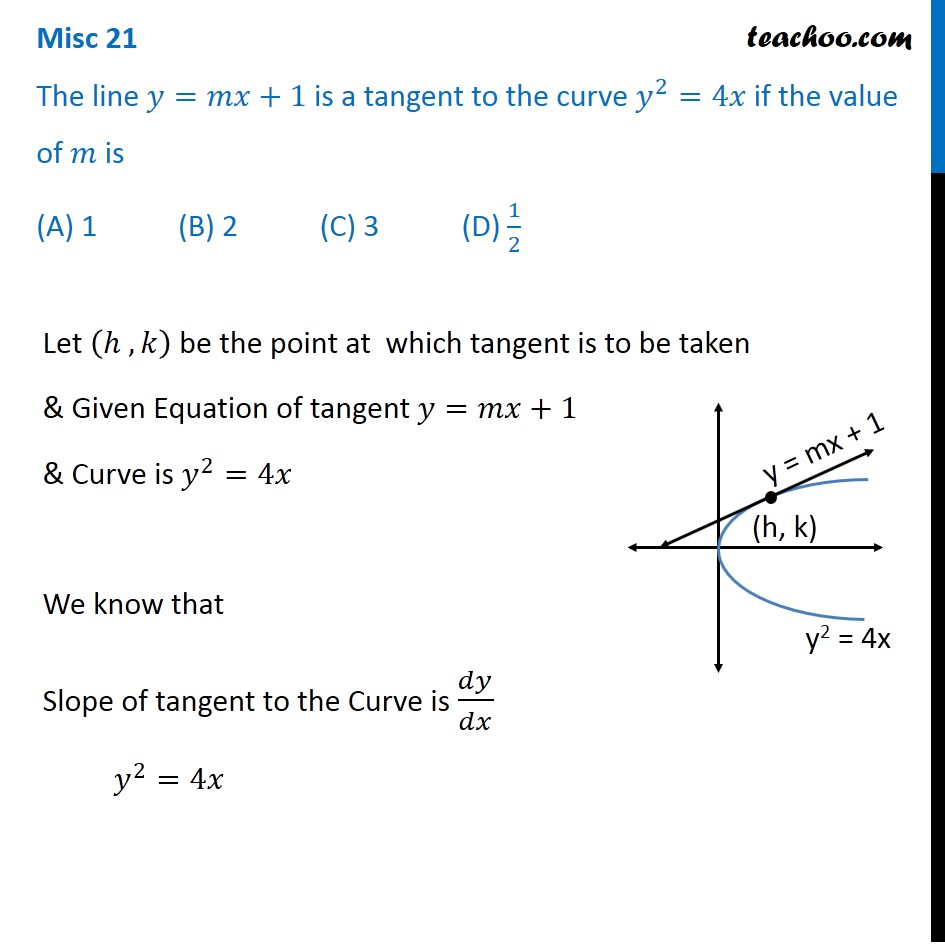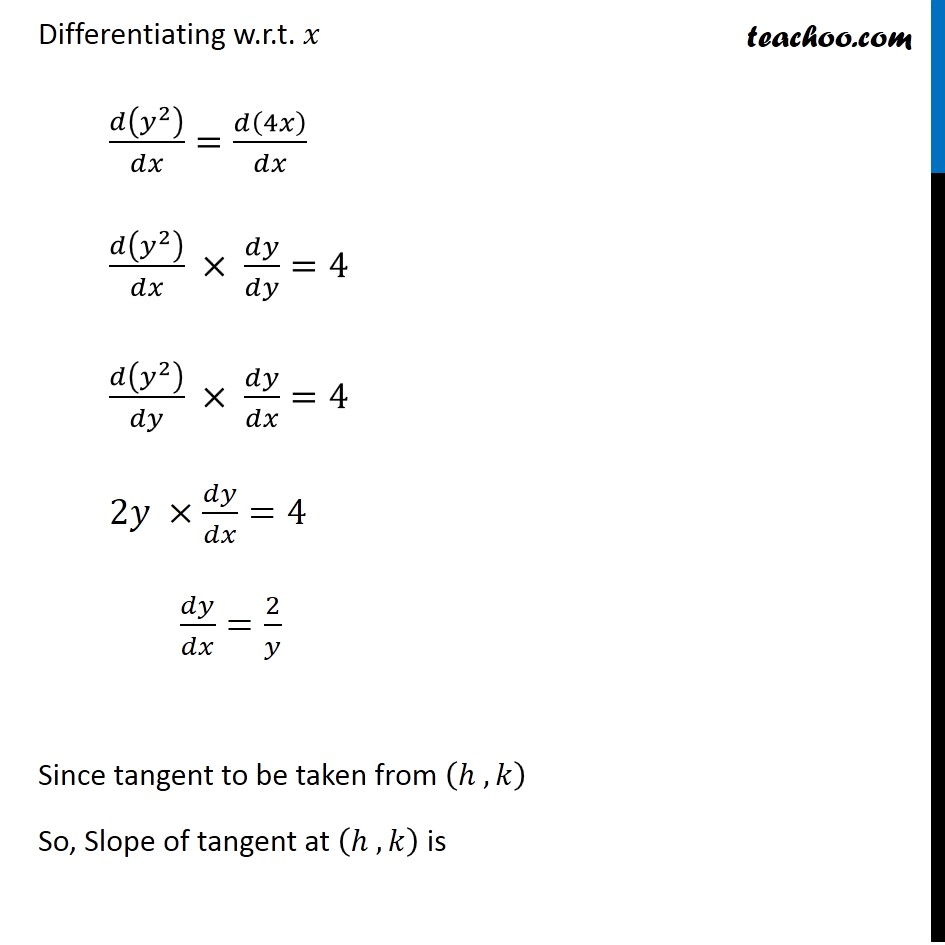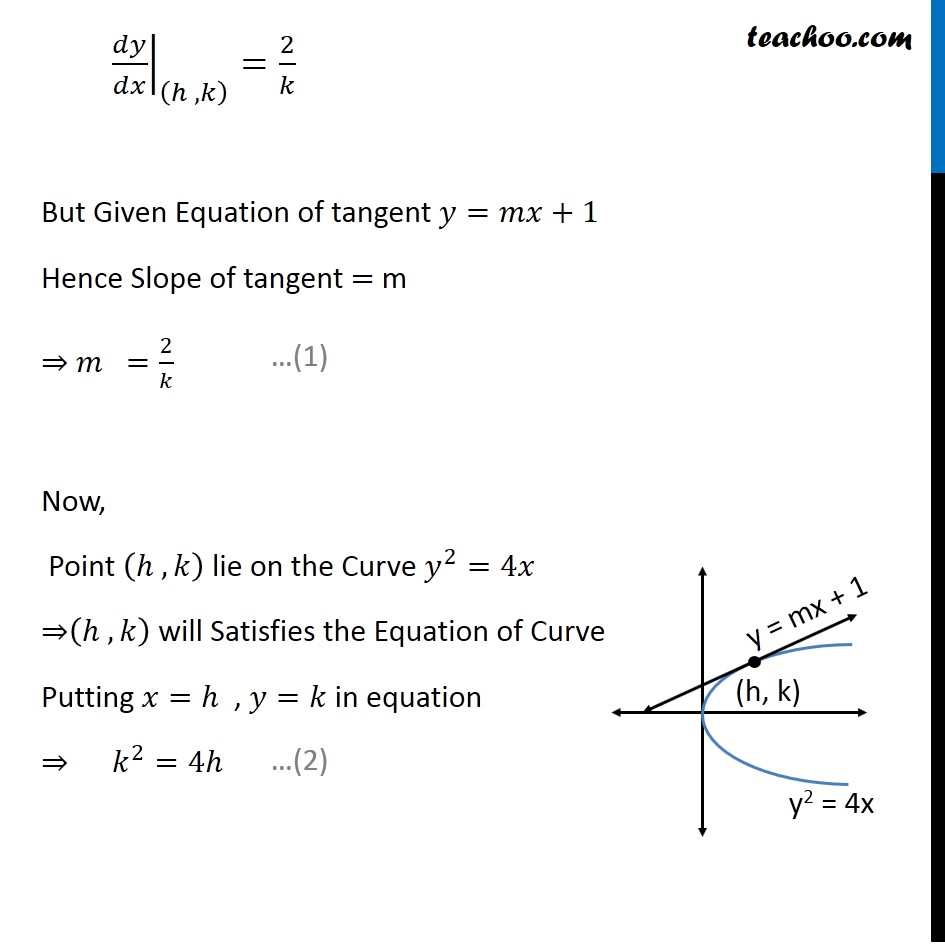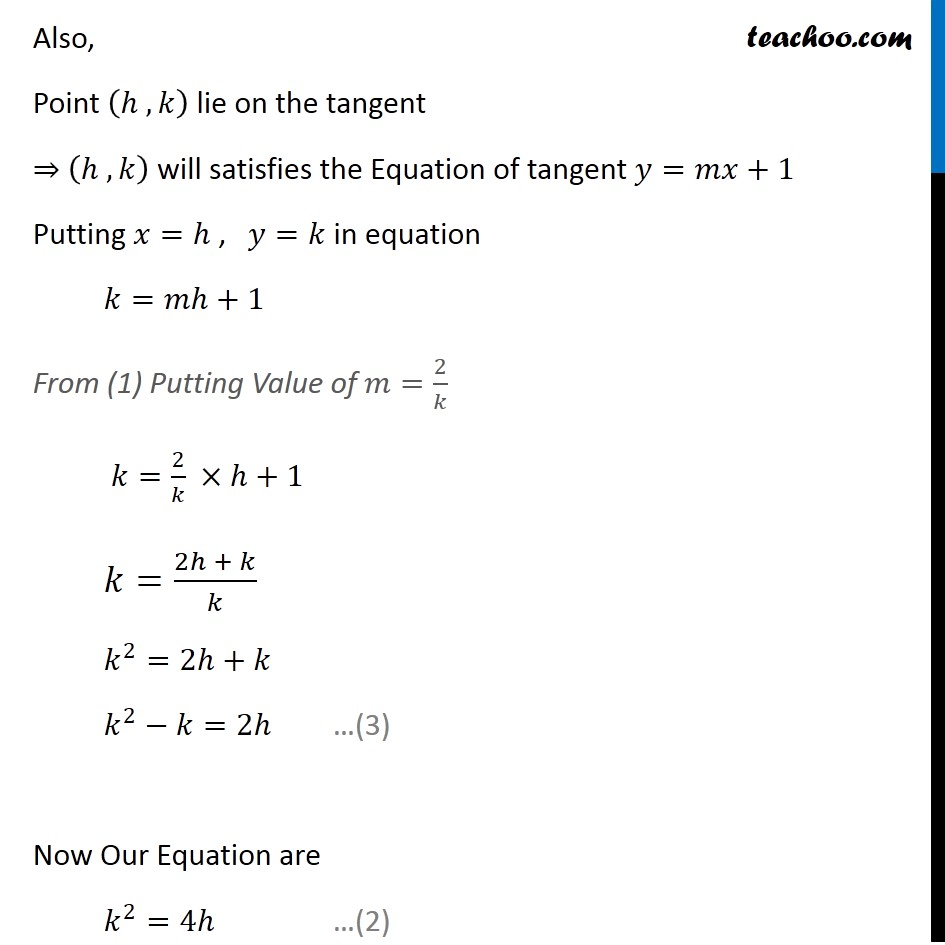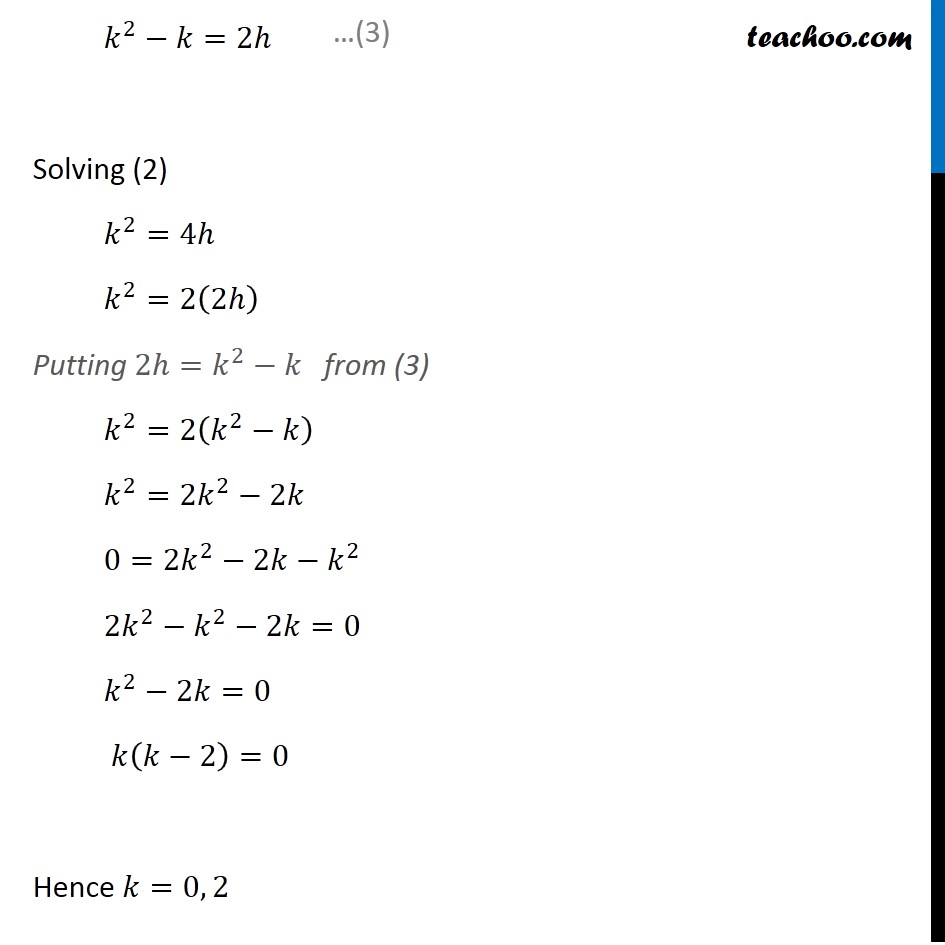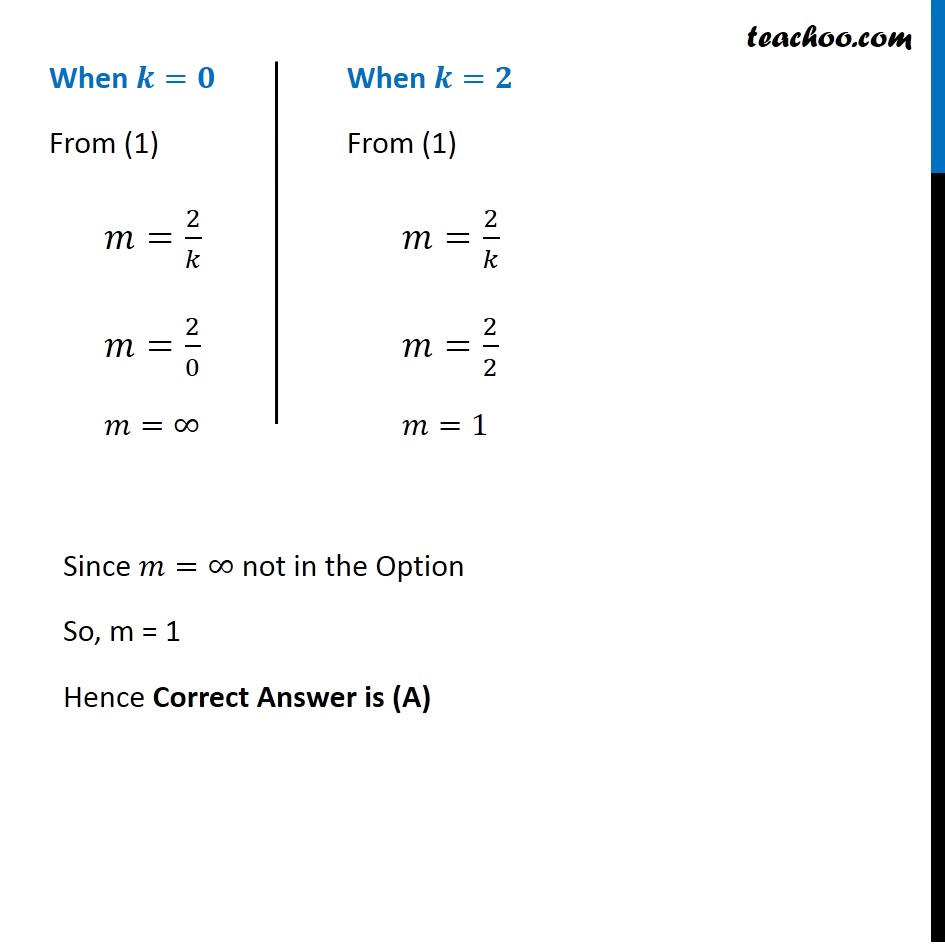1. Chapter 6 Class 12 Application of Derivatives
2. Serial order wise
3. Miscellaneous

Transcript

Misc 21 The line 𝑦=𝑚𝑥+1 is a tangent to the curve 𝑦^2=4𝑥 if the value of 𝑚 is (A) 1 (B) 2 (C) 3 (D) 1/2 Let (ℎ , 𝑘) be the point at which tangent is to be taken & Given Equation of tangent 𝑦=𝑚𝑥+1 & Curve is 𝑦^2=4𝑥 We know that Slope of tangent to the Curve is 𝑑𝑦/𝑑𝑥 𝑦^2=4𝑥 Differentiating w.r.t. 𝑥 𝑑(𝑦^2 )/𝑑𝑥=𝑑(4𝑥)/𝑑𝑥 𝑑(𝑦^2 )/𝑑𝑥 × 𝑑𝑦/𝑑𝑦=4 𝑑(𝑦^2 )/𝑑𝑦 × 𝑑𝑦/𝑑𝑥=4 2𝑦 ×𝑑𝑦/𝑑𝑥=4 𝑑𝑦/𝑑𝑥=2/𝑦 Since tangent to be taken from (ℎ , 𝑘) So, Slope of tangent at (ℎ , 𝑘) is ├ 𝑑𝑦/𝑑𝑥┤|_((ℎ , 𝑘) )=2/𝑘 But Given Equation of tangent 𝑦=𝑚𝑥+1 Hence Slope of tangent = m ⇒ 𝑚 =2/𝑘 Now, Point (ℎ , 𝑘) lie on the Curve 𝑦^2=4𝑥 ⇒(ℎ , 𝑘) will Satisfies the Equation of Curve Putting 𝑥=ℎ , 𝑦=𝑘 in equation ⇒ 𝑘^2=4ℎ …(1) …(2) Also, Point (ℎ , 𝑘) lie on the tangent ⇒ (ℎ , 𝑘) will satisfies the Equation of tangent 𝑦=𝑚𝑥+1 Putting 𝑥=ℎ , 𝑦=𝑘 in equation 𝑘=𝑚ℎ+1 From (1) Putting Value of 𝑚=2/𝑘 𝑘=2/𝑘 ×ℎ+1 𝑘=(2ℎ + 𝑘)/𝑘 𝑘^2=2ℎ+𝑘 𝑘^2−𝑘=2ℎ Now Our Equation are 𝑘^2=4ℎ …(3) …(2) 𝑘^2−𝑘=2ℎ Solving (2) 𝑘^2=4ℎ 𝑘^2=2(2ℎ) Putting 2ℎ=𝑘^2−𝑘 from (3) 𝑘^2=2(𝑘^2−𝑘) 𝑘^2=2𝑘^2−2𝑘 0=2𝑘^2−2𝑘−𝑘^2 2𝑘^2−𝑘^2−2𝑘=0 𝑘^2−2𝑘=0 𝑘(𝑘−2)=0 Hence 𝑘=0, 2 When 𝒌=𝟎 From (1) 𝑚=2/𝑘 𝑚=2/0 𝑚=∞ When 𝒌=𝟐 From (1) 𝑚=2/𝑘 𝑚=2/2 𝑚=1 Since 𝑚=∞ not in the Option So, m = 1 Hence Correct Answer is (A)

Miscellaneous